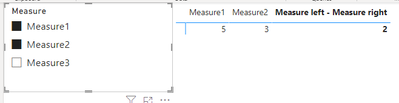cancel
Showing results for
Did you mean:Helper III

## how to get variance of selected measures from the slicers

Hey guys

Really want to explain straight - i have a slicer you can multi-select existing measures
suppose slicer is
* measure1
* measure2
* measure3

table shows how many measure is selected. Works fine !

user wants to see select two measures, and bring me those two measure's difference
suppose selected measures
* measure1
* measure2

expected view in the columns
measure1 | measure2 | (measure1-measure2) |

5        |        3        |                  2               |
and records below..

thanks!

1 ACCEPTED SOLUTIONCommunity Support

Hi @Bilhan ,

I suggest you to create two unrelated measure name tables to help calculation.

The code of "Measure Name" and "Measure selection" calculated tables are the same.

``````Measure Name =
DATATABLE (
"Measure", STRING,
"Sort", INTEGER,
{
{ "Measure1", 1 },
{ "Measure2", 2 },
{ "Measure3", 3 }
}
)``````

Data model:``````Measure Combine =
VAR _COMBINE =
'Measure Name',
"switch",
SWITCH (
'Measure Name'[Measure],
"Measure1", [Measure1],
"Measure2", [Measure2],
"Measure3", [Measure3]
)
)
VAR _LEFT =
SUMX ( FILTER ( _COMBINE, [Sort] = MIN ( 'Measure Selection'[Sort] ) ), [switch] )
VAR _RIGHT =
SUMX ( FILTER ( _COMBINE, [Sort] = MAX ( 'Measure Selection'[Sort] ) ), [switch] )
RETURN
IF (
ISFILTERED ( 'Measure Selection'[Measure] ),
IF (
HASONEVALUE ( 'Measure Name'[Measure] ),
SUMX (
FILTER (
_COMBINE,
'Measure Name'[Measure] IN VALUES ( 'Measure Selection'[Measure] )
),
[switch]
),
_LEFT - _RIGHT
),
"Please select two measures to compare"
)``````

Result is as below.Best Regards,
Rico Zhou

If this post helps, then please consider Accept it as the solution to help the other members find it more quickly.

2 REPLIES 2Helper III

@v-rzhou-msft
it works perfect except once you select three values it's calculation three of them and we don't want that
5| 3| 6 = -1 which I couldn't even find summarizing out of {5,3,6}Community Support

Hi @Bilhan ,

I suggest you to create two unrelated measure name tables to help calculation.

The code of "Measure Name" and "Measure selection" calculated tables are the same.

``````Measure Name =
DATATABLE (
"Measure", STRING,
"Sort", INTEGER,
{
{ "Measure1", 1 },
{ "Measure2", 2 },
{ "Measure3", 3 }
}
)``````

Data model:``````Measure Combine =
VAR _COMBINE =
'Measure Name',
"switch",
SWITCH (
'Measure Name'[Measure],
"Measure1", [Measure1],
"Measure2", [Measure2],
"Measure3", [Measure3]
)
)
VAR _LEFT =
SUMX ( FILTER ( _COMBINE, [Sort] = MIN ( 'Measure Selection'[Sort] ) ), [switch] )
VAR _RIGHT =
SUMX ( FILTER ( _COMBINE, [Sort] = MAX ( 'Measure Selection'[Sort] ) ), [switch] )
RETURN
IF (
ISFILTERED ( 'Measure Selection'[Measure] ),
IF (
HASONEVALUE ( 'Measure Name'[Measure] ),
SUMX (
FILTER (
_COMBINE,
'Measure Name'[Measure] IN VALUES ( 'Measure Selection'[Measure] )
),
[switch]
),
_LEFT - _RIGHT
),
"Please select two measures to compare"
)``````

Result is as below.Best Regards,
Rico Zhou

If this post helps, then please consider Accept it as the solution to help the other members find it more quickly.